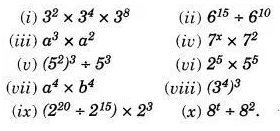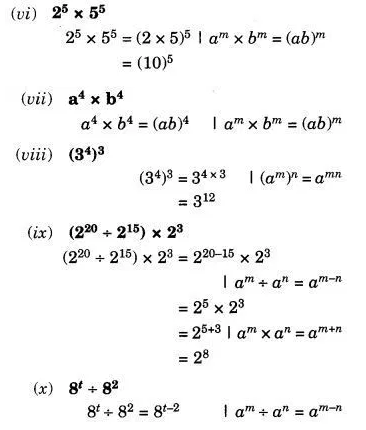# Using laws of exponents, simplify and write the answer in exponential form :

Using laws of exponents, simplify and write the answer in exponential form :